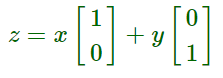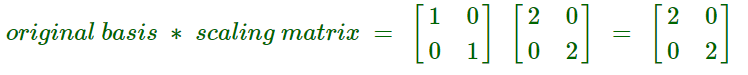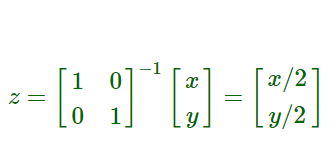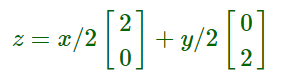Related Articles

# Differences between a Matrix and a Tensor

• Last Updated : 14 Jun, 2021

Matrix :
It is a tabular format in which numbers can be represented, like below –

Here, a11 means 1st row and 1st column. Rows are horizontal lines and columns are vertical lines. Here, the matrix is 4 x 4.
These are shown as n x m, where n is the number of rows and m is the number of columns.
Also called the 2-D arrays i.e. 2 Dimensional Arrays.

Basic mathematical operations can be done on matrices like Addition, Subtraction, Multiplication, but with some conditions. For + and -, the size of two matrices must be the same and for multiplication, it follows the below rule –

`(n X m) X (m X p) = n X p`

We can also multiply the entire matrix with a constant.
Example –

A =

B =

A  *  B  =

A matrix is like a simple BOX.
A matrix can be rectangular(nXm) or square(nXn).

Tensor :
Tensor is like a function, i.e. is linear in nature. It describes an object that is in space. Tensors are of different types –

1. 0 Rank tensors – Scalars
2. 1st Rank tensors – 1-D arrays
3. 2nd Rank tensors – 2-D arrays (A matrix)
4. nth Rank tensors – n-D arrays

So tensor is an n-dimensional array satisfying a particular transformation law. Unlike a matrix, it shows an object placed in a specific coordinate system. When the coordinate systems change, the entries of a tensor also transform in that way, such that the tensor still describes the same map in the new coordinate system.

Most machine learning algorithms use tensor to perform any calculation. In a defined system, a matrix is just a container for entries and it doesn’t change if any change occurs in the system, whereas a tensor is an entity in the system that interacts with other entities in a system and changes its values when other values change.

To understand this point, let’s see an example –
Assume there is a system having all matrices of 2×2 i.e. the coordinate system contains only 2 directions. Suddenly, a new direction came and we had to shift our matrices to 3×3. With matrices, this is not possible. Instead, if we use tensors, then by changing only 1 tensor, all other tensors will be changed to 3×3. This dynamism is not allowed in matrices.

The major difference is that a matrix has only 2 indices (can also be represented as M[n][m]) whereas tensors can have any indices( T[i1][i2][i3]….) even tensor can be a single number without any index.

To sum this in a single line we can say that, All matrices are not tensors, although all Rank 2 tensors are matrices.
Still confused let’s see an easy example of Rank 1 tensor.
Say this is our tensor in the standard Euclidean basis –Now, let’s shift to the basis of 2 using the following transformation rule –To do that, we will multiply it by the inverse of a scaling matrix –Now, after scaling, our tensor becomes –And yet it gives the same results.
This operation is not possible with matrices.
Moreover, in a matrix, each entity is a number. Example –

But in a tensor, each entity can be another tensor also. Example –

Similarly, we can have other degree tensors also.
The RGB Image can be represented by tensors having 3 layers of a 2D matrix.
A tensor can contain a matrix, but a matrix can’t contain a tensor.
Another definition of the tensor could be that it is a multi-way extension of a matrix.
Let’s see the product of a tensor –

T1 =

T2 =

T1 * T2 =

This is how tensors are multiplied.

My Personal Notes arrow_drop_up